Learning Library

Probability Darts

5th graders can practice calculating the probability that a dart will hit a given sector of a dart board using these fun probability worksheets.

Click on a worksheet in the set below to see more info or download the PDF.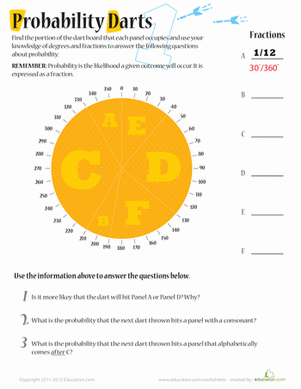Probability Darts 1

Kids will practice with fractions and degrees in this probability worksheet. Help your kid figure out the probability of a dart landing in different places.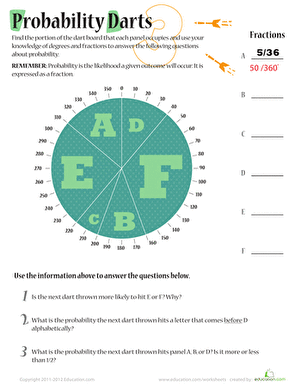Probability Darts 3

Build on fraction math skills and work with degrees to get probability practice for your middle schooler with this math worksheet series.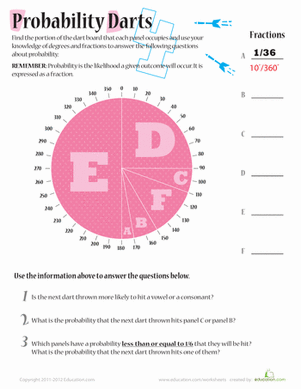Probability Darts 4

Building on math skills with fractions, addition, and degrees, kids will figure out how much of the dart board each panel takes up then answer questions.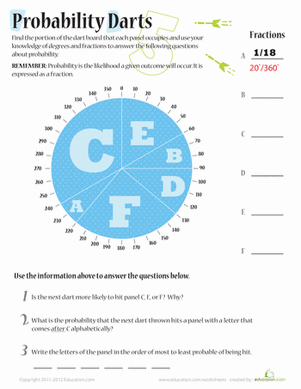Probability Darts 5

Build on fraction math skills and working with degrees up to 360° get probability practice for your middle schooler with this math worksheet series.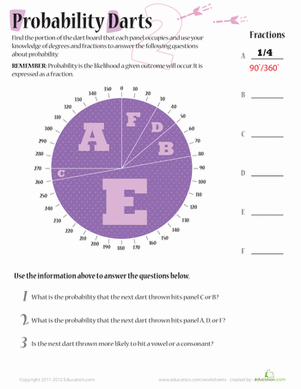Probability Darts 2

Build on fraction math skills and working with degrees to get probability practice for your middle schooler with this math worksheet series.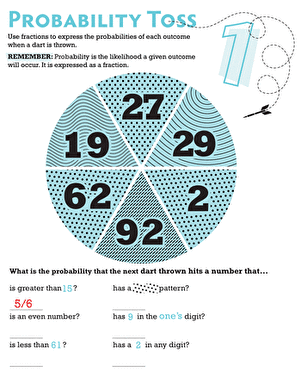Probability Toss 1

What are the chances that a dart will land on an even number on a dart board? Find out in this sixth grade probability worksheet!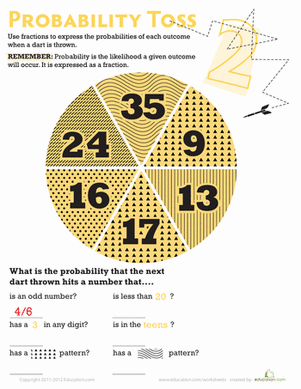Probability Toss 2

Boost your child's math know-how! What are the odds a dart would land on one of the given sections of this circle?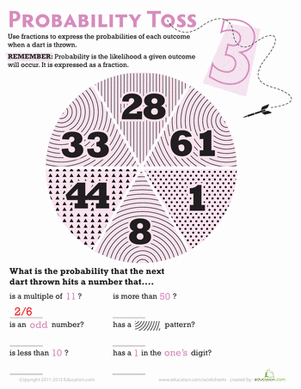Probability Toss 3

If your sixth grader likes darts, then he'll love this probability worksheet! This dart board themed worksheet introduces your child to simple probability.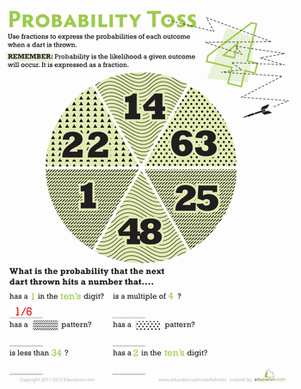Probability Toss 4

Help your sixth grader learn about probability with a dart board math worksheet. Use math skills to calculate the probability of a dart hitting number types.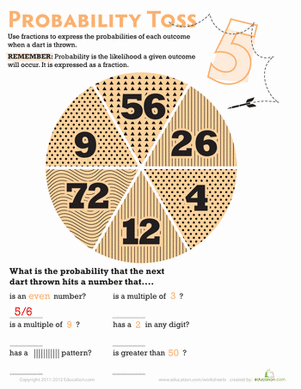Probability Toss 5

Sixth graders will practice probability in this dart board themed worksheet. Your kid will calculate the probability of a dart hitting a certain kind of number.

Want to download this whole set as a single PDF?

Create new collection

0

New Collection>

0 items

What could we do to improve Education.com?

Please note: Use the Contact Us link at the bottom of our website for account-specific questions or issues.

What would make you love Education.com?